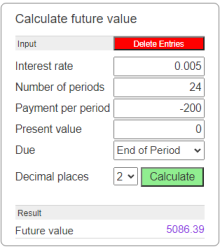# FV Function (future value)

Returns a value specifying the future value of an annuity

## Description of the function FV

The FV function Returns a value specifying the future value of an annuity based on periodic, fixed payments and a fixed interest rate.

An annuity is a series of fixed cash payments made over time. An annuity can be a loan (such as a home mortgage) or an investment (such as a monthly savings plan).

The Rate and NPer arguments must be calculated using payment periods expressed in the same units. For example, if Rate is calculated using months, NPer must also be calculated using months.

For all arguments, cash paid out (such as deposits to savings) is represented by negative numbers; cash received (such as dividend checks) is represented by positive numbers.

Calculate future value

 Input Interest rate Number of periods Payment per period Present value Due End of Period Begin of Period Decimal places 0 1 2 3 4 6 Result Future value

#### Example## Description of the parameters

Interest rate

The interest rate per period. For example, if you get a car loan at an annual percentage rate (APR) of 10 percent and make monthly payments, the rate per period is 0.1/12, or 0.0083.

Number of periods

The total number of payment periods in the annuity. For example, if you make monthly payments on a four-year car loan, your loan has a total of 4 x 12 (or 48) payment periods.

Payment of each period

The payment to be made each period. Payments usually contain principal and interest that doesn't change over the life of the annuity.

Present value

The present value (or lump sum) of a series of future payments. For example, when you borrow money to buy a car, the loan amount is the present value to the lender of the monthly car payments you will make. The default is 0.

Due

Due date specifies when payments are due. This argument must be either End of Period if payments are due at the end of the payment period, or Begin of Period if payments are due at the beginning of the period.